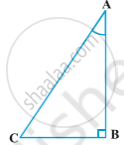SSC (Marathi Semi-English) 10thMaharashtra State Board
Share

Books Shortlist

# Concept: Trigonometric Identities

#### notes

You may recall that an equation is called an identity when it is true for all values of the variables involved. Similarly, an equation involving trigonometric ratios of an angle is called a trigonometric identity, if it is true for all values of the angle(s) involved.In ∆ ABC, right-angled at B, we have;

"AB"^2 + "BC"^2 = "AC"^2      (1)

Dividing each term of (1) by "AC"^2, we get

"AB"^2/"AC"^2 +"BC"^2/"AC"^2= "AC"^2/"AC"^2

("AB"/"AC")^2 + ("BC"/"AC")^2= ("AC"/"AC")^2

(cos A)^2 + (sin A)^2 = 1

cos^2 A + sin^2 A = 1         (2)

(i) cos^2 A = 1- sin^2 A

(ii) sin^2 A = 1-cos^2 A

This is true for all A such that 0° ≤ A ≤ 90°. So, this is a trigonometric identity.

Let us now divide (1) by "AB"^2. We get

"AB"^2/"AB"^2 + "BC"^2/"AB"^2= "AC"^2/"AB"^2

("AB"/"AB")^2 + ("BC"/"AB")^2= ("AC"/"AB")^2

1+tan^2A= sec^2A         (3)

(i) tan^2A= sec^2A-1

(ii)sec^2A- tan^2A= 1

This is true for all A such that 0° ≤ A < 90°.

Let us see what we get on dividing (1) by "BC"^2. We get,

"AB"^2/"BC"^2 +"BC"^2/"BC"^2= "AC"^2/"BC"^2

("AB"/"BC")^2 + ("BC"/"BC")^2= ("AC"/"BC")^2

cot^2A+1= cosec^2A      (4)

(i)cot^2 A= cosec^2 A- 1

(ii)cosec^2 A- cot^2 A= 1

Example 1 : Express the ratios cos A, tan A and sec A in terms of sin A.

Solution : Since cos^2 A + sin^2 A = 1, therefore,

cos^2 A = 1 – sin^2 A, i.e., cos A = +or- sqrt(1-sin^2A)

This gives cos A = sqrt(1-sin^2A)

Hence, tanA= "sinA"/"cosA"= "sinA"/sqrt(1-sin^2A) and

sec A= 1/"cosA"= 1/sqrt(1-sin^2A)

S